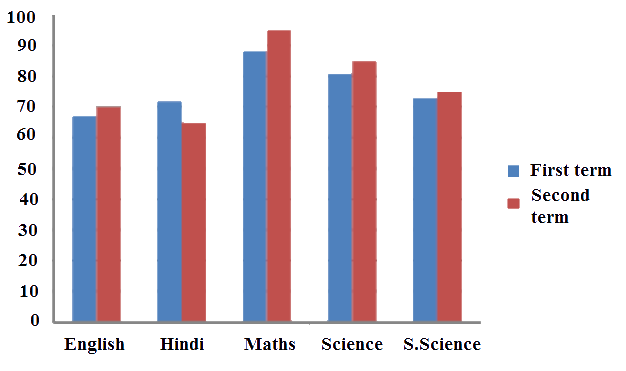# Ex.3.3 Q4 Data Handling - NCERT Maths Class 7

Go back to  'Ex.3.3'

## Question

The performance of a student in $$1^{st}$$ term and $$2^{nd}$$ term is given. Draw a double bar graph choosing appropriate scale and answer the following:

 Subject English Hindi Maths Science S. Science $$1^{st}$$ Term (M.M. $$100$$) $$67$$ $$72$$ $$88$$ $$81$$ $$73$$ $$2^{nd}$$ Term (M.M. $$100$$) $$70$$ $$65$$ $$95$$ $$85$$ $$75$$

(i) In which subject, has the child improved his performance the most?

(ii) In which subject improvement is the least?

(iii) Has the performance gone down in any subject?

Video Solution
Data Handling
Ex 3.3 | Question 4

## Text Solution

What is known?

Marks obtained in different subject

Reasoning:

We have to draw the graph by using the data

Steps:Difference between the marks of $$1^{st}$$ and $$2^{nd}$$ term

English $$= 70 \, – 67 = 3$$

Hindi $$= 65 \,– 72 = -7$$ (Decrease in marks)

Math $$= 95\, – 88 = 7$$

Science $$= 85\, – 81= 4$$

Social Science $$= 75\, – 73 =2$$

(i) In Math, the performance of the students improved.

(ii) In Social .science, the performance of the students improved is the least.

(iii)Yes, in Hindi the performance of the students has gone down.

Learn from the best math teachers and top your exams

• Live one on one classroom and doubt clearing
• Practice worksheets in and after class for conceptual clarity
• Personalized curriculum to keep up with school